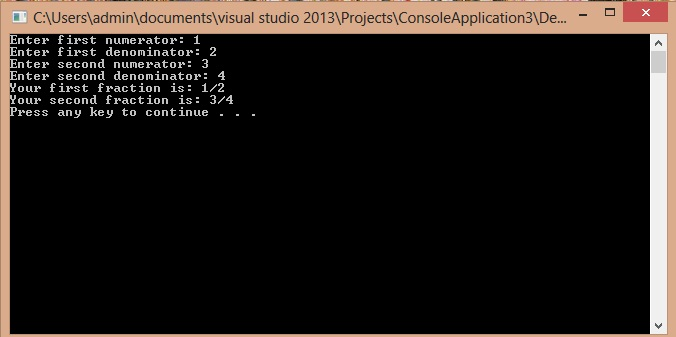# Getting Started With Object Oriented Programming

In this part you will learn: 1. Why we need Object Oriented Programming approach? 2. Classes 3. Access specifiers 4. First program using Classes 5. Dot operator 6. Showing output Why we need Object Oriented Programming approach? As we have already learned in c how to perform different operations and process information, using that ways we can write small programs but if we are writing programs for very large projects this approach isn’t really a good one. The programs may work correct but it would be really a tough job debugging (removing errors) from that code as there will be numerous variables, arrays, functions. Moreover we see that in most of the cases we are using the same kind of variables and functions for different data. So in c++, we have a programming approach called Object Oriented Programming which helps us making our tasks easy. What is a Class? In Object oriented programming, class is the heart of it. Everything related to object oriented programming and even data structures revolves around it. There are different variables in a class called member variables and the functions to perform different operation on them are called member functions of that particular class. Classes make writing, debugging and maintaining a program easy. If you want to make changes in a program you know exactly which function to make changes in. It will make that change for all the objects made by that type. A class and an object of that class has the same relationship as a data type and a variable. Access specifiers The last thing to know about before writing our first program on the basis of class is Access specifiers. As the name suggests Access specifier mean specifying the type of access to different member variables and function. There are three types of Access specifiers. • Private • Protected • Public The most commonly used access specifiers are private and public but we will discuss all of them in detail later. For now just keep in mind that Public gives access to all the member variables and functions to the programmer using the object of that class whereas if we use private as our access specifier, it will hide all the member variables and functions under it. Syntax Following is the basic syntax of class definition
`class class_name{Access specifier:Member functions or variables or bothAccess specifier:Member functions or variables or both}`
Our first program using class In our first example we will write a program to get numerator and denominator of two different fractions from the user and store them in num and denom of two different objects of a class namely “fraction”. Then we will print them on the screen. Note: In this program we will use “public” as our access specifier which gives access to the members under it outside the class. Basic Step:
`#include<iostream>#include<conio.h>using namespace std;`
These two are the most common and basic lines of a c++ program. The first one iostream is used to grant us most of the basic functions of C++ like Input and Output functions. The second one conio.h means console input output, the black screen we see is the console. Using namespace std; provides us an ease of using all the library functions without specifying that this function belongs to standard library. Without including it, we have to write ‘std’ with all the built-in functions .
`class fraction					{public:			//access specifier 				int num;	int denom;}; int main(){	fraction f1;		//initializing a variable f1 of type fraction	fraction f2;		//initializing a variable f2 of type fraction	cout << "Enter first numerator: ";        cin >> f1.num;   	//note that we are using the member num of   fraction f1	cout << "Enter first denominator: ";        cin >> f1.denom; 	//note that we are using the member denom of fraction f1	cout << "Enter second numerator: ";	cin >> f2.num;	cout << "Enter second denominator: ";	cin >> f2.denom; 	cout<<"Your first fraction is: " << f1.num << "/" << f1.denom << endl;	cout<<"Your second fraction is: " << f2.num << "/" << f2.denom <<  endl; 	system("pause");}`
In this program, at first we have initialized a class namely “fraction”. Then in the curly brackets we have first specified the type of access specifier and then given the names of the member variables of our class i.e num and denom and their types are integers. Since no other variable is required we will move towards the usage of this class in the main program. Note: It is necessary to use semi-colon after closing bracket of our class as it is the part of syntax In our main program we will initialize two variables namely f1 and f2 of data type “fraction”. This is the most important part. Variables of class type (objects) are initialized just like other built-in data types (int,char,double,float). That means when we write fraction f1; it means that we have initialized a variable f1 of type fraction which has two members namely num and denom. f1 and f2 will be two separate variables num and denom of their own. Then we will ask the user to enter the value of numerator of the first fraction. As you can see that it’s the numerator of the first fraction so we will store this numerator in the “num” of “f1”. For this purpose we will use dot operator. Dot operator A dot operator has the object name on its left while the right side has the member name which we want to access. Since it is a public member, we have direct access to it. In the above code we have written “cin >> f1.num;” which means save the value obtained from console in the member “num” of fraction “f1”. After that we will do the same to get denom of first fraction “f1”. Then we will take num and denom of “f2” from the user. Finally we will print both fractions f1 and f2 using their seperate member variables num and denom. Output